#### 期刊菜单

Dynamic Response Analysis of Vehicle-Pier Collision with Multi Parameters
DOI: 10.12677/HJCE.2022.1111134, PDF , HTML, XML, 下载: 103  浏览: 188  国家自然科学基金支持

Abstract: Longitudinal slope along the bridge, horizontal impact angle and horizontal eccentricity are the main geometric parameters of vehicle-pier collision. The influence of these parameters on vehicle-pier collision dynamic response under different vehicle speeds is studied. Based on the simplified vehicle model, a three-dimensional dynamic finite element model for vehicle-pier collision simulation is established by using ANSYS and LS-DYNA software. Taking a square pier as an example, the effects of the geometric parameters on the impact force along the bridge when the speed is 40 Km/h, 60 Km/h, 80 Km/h are analyzed. The results show that the maximum impact force along the bridge increases with the increase of impact speed. At the same speed, as the slope increases, the maximum impact force along the bridge shows a decreasing trend. With the increase of horizontal impact angle, the maximum impact force along the bridge shows a decreasing trend. When the horizontal eccentricity is less than 0.8 m, the maximum of the impact force along the bridge increases with the increase of the horizontal eccentricity. When the horizontal eccentricity is 0.8 m, the peak value of impact force along the bridge reaches the maximum value. When the horizontal eccentricity is greater than 0.8 m, the peak value of impact force along the bridge decreases with the increase of horizontal eccentricity. The research points out the trend of the relationship between the geometry parameters and the peak value of impact force, which can provide a theoretical basis for bridge collision prevention decision and design.

1. 引言

2. 基于弹塑性随动硬化模型和刚体模型的有限元建模思路

2.1. 车辆简化模型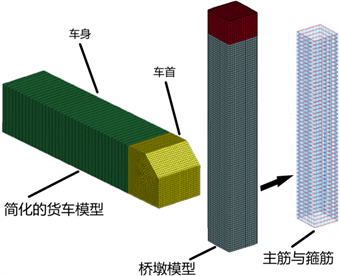Figure 1. Finite element model of the vehicle-pier collisionTable 1. MAT_PLASTIC_KINEMATIC material parameters

2.2. 桥墩模型Table 2. HJC model material parameters

2.3. 接触定义

3. 动力响应分析

3.1. 桥梁纵坡对车桥碰撞的动力响应影响分析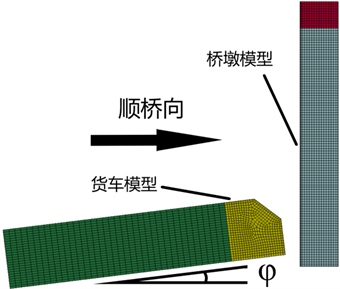Figure 2. Schematic diagram of longitudinal slope along the bridge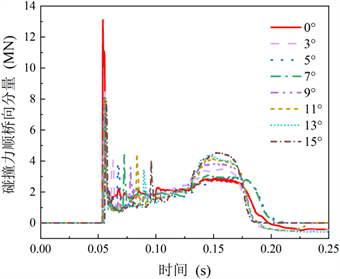Figure 3. Time history curves of impact forces along the bridge at different longitudinal slope along the bridge (vehicle speed = 40 km/h)

3.2. 碰撞力水平偏角对车桥碰撞的动力响应影响分析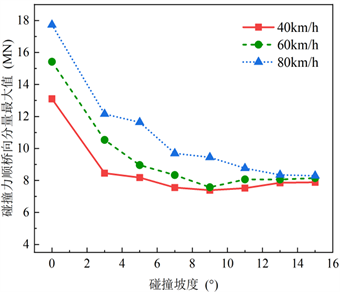Figure 4. Relationship between longitudinal slope along the bridge and maximum impact force along the bridge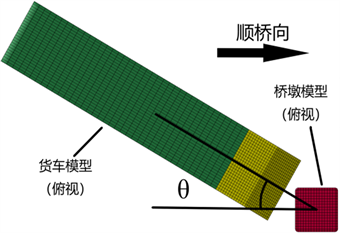Figure 5. Schematic diagram of horizontal impact angle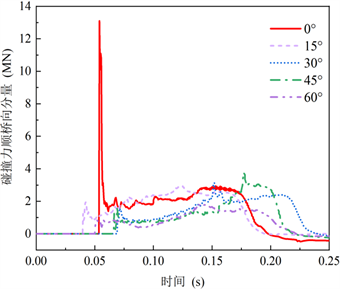Figure 6. Time history curve of impact force along the bridge under different horizontal impact angle (vehicle speed = 40 km/h)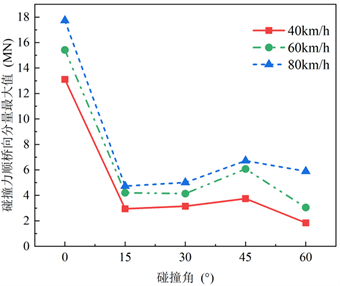Figure 7. Relationship between horizontal impact angle and maximun impact force along the bridge

3.3. 撞击偏心距对车桥碰撞的动力响应影响分析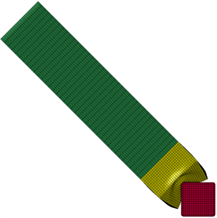Figure 8. Deformation of the vehicle when horizontal impact angle is 45˚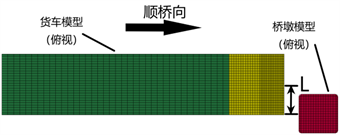Figure 9. Schematic diagram of horizontal eccentricity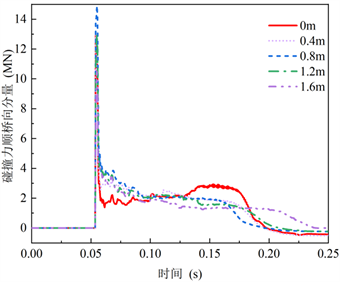Figure 10. Time history curve of impact force along the bridge under different horizontal eccentricity (vehicle speed = 40 km/h)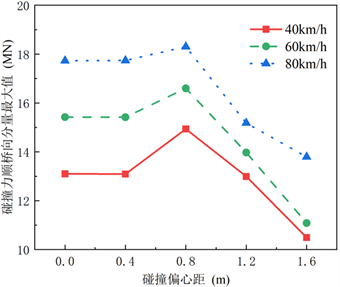Figure 11. Relationship between horizontal eccentricity and maximun impact force along the bridge

4. 结论与展望

1) 碰撞时的汽车速度是影响车桥碰撞动力响应的因素之一，顺桥向撞击力最大值随汽车速度的增加而增加；

2) 就同一碰撞速度而言，随着碰撞纵坡(碰撞竖向偏角)增大，顺桥向碰撞力最大值呈现下降趋势；

3) 就同一碰撞速度而言，随着碰撞力水平偏角增加，顺桥向碰撞力最大值呈现下降趋势。

  王潇宇, Demartino, C., 徐金俊, 肖岩. 侧向冲击作用下钢管混凝土柱动力响应试验研究及计算方法[J]. 土木工程学报, 2017, 50(12): 28-36.  Sohel, K.M.A., Al-Jabri, K. and Al Abri, A.H.S. (2020) Behavior and Design of Reinforced Concrete Building Columns Subjected to Low-Velocity Car Impact. Structures, 26, 601-616. https: //doi.org/10.1016/j.istruc.2020.04.054  李瑞文, 周德源. 车辆撞击下桥墩动力响应与撞击荷载研究[J]. 结构工程师, 2019, 35(2): 83-92.  Yao, P., Zhu, J., Zhu, L., Fang, H. and Qian, C. (2021) Experimental and Numerical Analysis of Hollow and Solid Reinforced Concrete Piers under Static and Impact Loadings. Shock and Vibration, 2021, Article ID: 9926010. https: //doi.org/10.1155/2021/9926010  Li, R.W., Zhou, D.Y. and Wu, H. (2020) Experimental and Numerical Study on Impact Resistance of RC Bridge Piers under Lateral Impact Loading. Engineering Failure Analysis, 109, Article ID: 104319. https: //doi.org/10.1016/j.engfailanal.2019.104319  赵武超, 钱江, 侧向冲击截载下钢筋混凝土墩柱的性能[J]. 工程科学学报, 2019, 41(3): 408-415.  曹伟, 吴合良, 贺耀北. 车撞作用下桥墩非线性损伤及加固措施研究[J]. 中外公路, 2017, 37(4): 147-151.  Zhao, W. and Ye, J. (2021) Impact Force Characteristic and Mechanical Behavior of Trucks in Collisions against Bridge Piers. Structures, 34, 1489-1506. https: //doi.org/10.1016/j.istruc.2021.08.049  康昌敏, 王蕊, 朱翔. 轴压比对钢管混凝土柱侧向冲击性能影响研究[J]. 工程力学, 2020, 37(Z1): 254-260.  司强, 王蕊. 冲击荷载下内衬八边形钢管空心钢筋混凝土柱的动力响应[J]. 爆炸与冲击, 2019, 39(11): 85-94.  陈林, 曾玉烨, 颜泽峰, 祝明桥. 车辆撞击下钢筋混凝土桥墩的动力响应及损伤特征[J]. 振动与冲击, 2019, 38(13): 261-267, 273.  Peng, K.K. (2021) Dynamic Ship-Bridge Collision Risk Decision Method Based on Time-Dependent AASHTO Model. Journal of the Institution of Engineers (India): Series A, 102, 305-313. https: //doi.org/10.1007/s40030-020-00506-9  Heng, K., Li, R., Li, Z. and Wu, H. (2021) Dynamic Responses of Highway Bridge Subjected to Heavy Truck Impact. Engineering Structures, 232, Article ID: 111828. https: //doi.org/10.1016/j.engstruct.2020.111828  王建国, 陈涛. 船舶与大跨度斜拉桥碰撞的有限元数值模拟[J]. 桥梁建设, 2016, 46(5): 12-17.  Chen, X., Xu, L. and Zhu, Q. (2017) Mechanical Behavior and Damage Evolution for Concrete Subjected to Multiple Impact Loading. KSCE Journal of Civil Engineering, 21, 2351-2359. https: //doi.org/10.1007/s12205-016-1143-8  Heng, K., Jia, P., Xu, J., Li, R. and Wu, H. (2022) Vehicular Impact Resistance of Highway Bridge with Seismically-Designed UHPC Pier. Engineering Structures, 252, Article ID: 113635. https: //doi.org/10.1016/j.engstruct.2021.113635